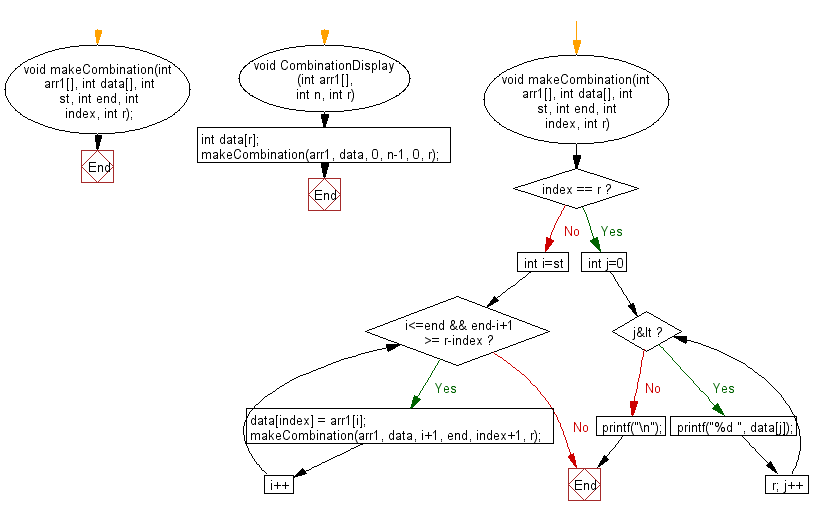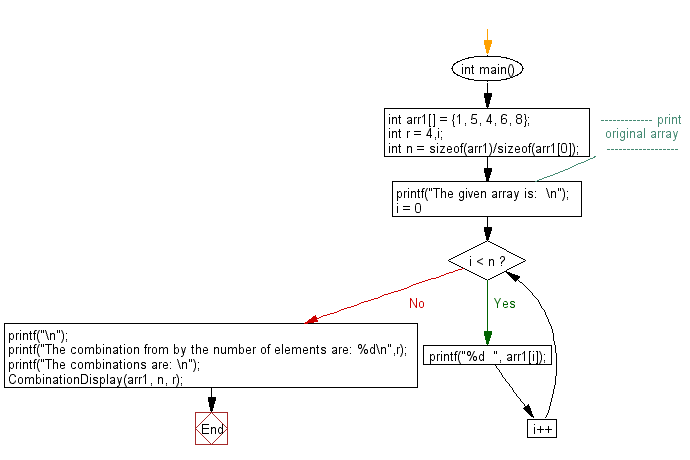﻿ C exercises: Print all possible combinations of r elements in a given array - w3resource# C Exercises: Print all possible combinations of r elements in a given array

## C Array: Exercise-82 with Solution

Write a program in C to print all possible combinations of r elements in a given array.

Sample Solution:

C Code:

``````#include <stdio.h>
void makeCombination(int arr1[], int data[], int st, int end,
int index, int r);

void CombinationDisplay(int arr1[], int n, int r)
{
int data[r];
makeCombination(arr1, data, 0, n-1, 0, r);
}

void makeCombination(int arr1[], int data[], int st, int end,
int index, int r)
{

if (index == r)
{
for (int j=0; j<r; j++)
printf("%d ", data[j]);
printf("\n");
return;
}
for (int i=st; i<=end && end-i+1 >= r-index; i++)
{
data[index] = arr1[i];
makeCombination(arr1, data, i+1, end, index+1, r);
}
}
int main()
{
int arr1[] = {1, 5, 4, 6, 8};
int r = 4,i;
int n = sizeof(arr1)/sizeof(arr1);
//------------- print original array ------------------
printf("The given array is:  \n");
for(i = 0; i < n; i++)
{
printf("%d  ", arr1[i]);
}
printf("\n");
//------------------------------------------------------
printf("The combination from by the number of elements are: %d\n",r);
printf("The combinations are: \n");
CombinationDisplay(arr1, n, r);
}
```
```

Sample Output:

```The given array is:
1  5  4  6  8
The combination from by the number of elements are: 4
The combinations are:
1 5 4 6
1 5 4 8
1 5 6 8
1 4 6 8
5 4 6 8
```

Pictorial Presentation:Flowchart 1:Flowchart 2:C Programming Code Editor:

Improve this sample solution and post your code through Disqus.

﻿

## C Programming: Tips of the Day

Where is the C auto keyword used?

auto is a modifier like static. It defines the storage class of a variable. However, since the default for local variables is auto, you don't normally need to manually specify it.

Ref : https://bit.ly/3yzwC9r